Inverse Kinematics

Lets recap what is Forward kinematics first.

Forward kinematics is the problem of finding the position and orientation of the end-effector, given all the joint parameters.

Inverse kinematics is simply the reverse problem i.e., given the target position and orientation of the end-effector, we have to find the joint parameters.

For example we have a kinematic chain with n joints as shown in fig 1. Lets say q1,  q2,  q3, ....... qn  are the joint variables.

Now we are given with the target position and orientation of the end effector i.e., Transform matrix Tb-ee from base to end-effector. Now our task is to find the joint variables   q1,  q2,  q3, ........ qn .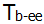Fig. 1 Example of Inverse Kinematics problem

Target Pose: Tb-ee

Known Values: x, y, z, R11, R12, R13,....... R33, link lengths (L1, L2,.... Ln)

To Find: q1, q2, q3,...... qnThis is the inverse kinematics problem.

Inverse kinematic is the tougher problem when compared to forward kinematics. Since this is tougher, mathematicians have come up with different approaches to solve this problem.

These approaches are mainly divided into two types.

1. Analytical solutions.

2. Approximate Solutions.

Analytical Solutions:

In Analytic solution to an inverse kinematics problem, we have a closed-form expression which gives you the inverse kinematics (joint variables) as a function of the end-effector pose. Which means we have a definite equations for each joint parameter. We just have to put the known values in the equations (like end-effector's target pose, robot link lengths) to get the joint parameters required to achieve that target posewhere,

q's are the joint parameters

f(x) are the equation in terms of known values (position and orientation of end effector, link lengths)

Few approaches in Analytical Solutions are

• Algebraic Approach
In this approach, we use the equations derived by equating the give Transformation matrix [target position and orientation] and the obtained Forward kinematics matrix of the robot. Using this equations, we will calculate the joint parameters q1,  q2,  q3, ........ qn .

For better understanding go through example1.

• Geometric Approach
In this approach, we will derive the trigonometric equations [using sine and cosine rules] by observing the physical structure of the robot/manipulator. By solving this equations we will calculate the joint parameters q1,  q2,  q3, ........ qn .

For better understanding go through example2.

Approximate Solutions:

Approximate solutions are also known as numerical solutions. We numerically compute the joint angles corresponding to an end-effector pose which means we do more than just plug in some numbers into an expression. Approximate solution typically rely on iterative optimization i.e., the target pose is reached by moving closer to it at each iteration.

Few Approaches in Approximate solutions are

• Jacobian Inverse technique
This is the most widely used method to solve the inverse kinematics problem. In this method we have to compute the Jacobian matrix and invert it. Check more on Jacobian Inverse technique here.

• Cyclic Co-ordinate descent
CCD solves the IK problem through
optimization. Looping through the joints from end to root, we optimize each joint to get the end effector (tip of the final joint) as close to target as possible. This loop is then repeated until we reach the target pose.

For better understanding on how CCD works, check this video.

Analytical solution Vs Approximate solution:Types of Robots:

Robots are classified into three types based on the ability to reach a given target pose (position and orientation).

Under-actuated Manipulators:

A manipulator with less than 3 DOF in 2D Space / 6-DOF in 3D Space are under-actuated. This type of robots cannot achieve all given arbitrary poses in the workspace. Under-actuated manipulator can reach a target position but it may not achieve the target orientation within the workspace.

Fully Actuated Manipulators:

A manipulator with exactly 3 DOF in 2D Space / 6-DOF in 3D Space are fully actuated. This type of robots can achieve all given arbitrary poses in the workspace. Sometimes there could be more than one possible ways to achieve the target pose within the workspace.

Redundant Manipulators:

A manipulator with more than 3 DOF in 2D Space / 6-DOF in 3D Space are redundant manipulators. This type of robots can achieve all given arbitrary poses in the workspace. There could be infinite possible ways to achieve the target pose within the workspace.That's all for the theory part on the inverse kinematics. Now its time to solve some real problems on inverse kinematics.

WARNING...!! WARNING...!!

Matrix multiplications and Trigonometric equations ahead.I know they are boring and may make you fall asleep. So take a break, have a cup of coffee and go ahead.

Example 1:

In this example we will solve the inverse kinematics problem of a RRR planar manipulator, shown in fig 2, using Algebraic Approach.Fig. 2 2 Link RRR planar manipulator

The first step in this method is to find the Forward kinematic equations of the robot.

So lets find FK first.Fig. 3 Frames attached to manipulator to find FK

As you can see in the fig 3, the base frame {b} and the frame attached to link1 {1}, are at the same point. Base frame is a fixed frame and frame {1} rotates about joint1.

Now lets find the transformations of the adjacent frames Tb1, T12, T2-ee.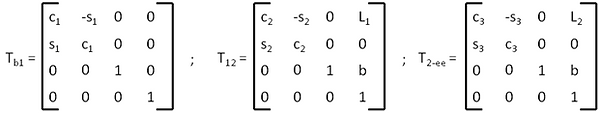The next step is to find the transformation from base to end-effector.The transformation of any planar manipulator can be represented as below,For the inverse kinematics problem of a planar manipulator, you are given with target position (x, y) and target orientation ψ.

In our RRR manipulator have 3 joint variables q1,  q2,  q3 . So we need 3 equations to solve IK. Come lets find the 3 equations.

From (1) and (2), we can writeNote: The target orientation of the end-effector is equal to the sum of all revolute joint angles in planar manipulator.

Also we can write target orientation ψ as below,Now we have 3 equations (a), (b) and (c). Therefore we can find the joint parameters q1,  q2,  q3 by solving these equations.

Lets start solving,

Square and Add (a) and (b).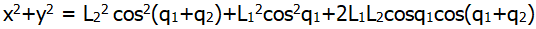Therefore,Since we got q2 , we can substitute this value in equ (b) and find q1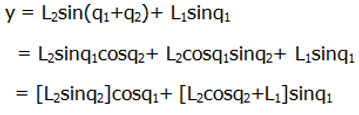The above equation is in the form of acosx+bsinx=c. When in this form, x can be written as below,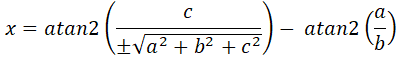Therefore, we can write q1 as below,From equ (d), we can rewrite the above equation as below,Now using equ (c) we can find q3Lets write all three equations together,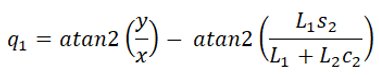If you see q2, there can be two possible values based on x and y values. Henceforth two solutions for same target pose as shown in fig 4. As we discussed in "Types of Robots", this is a fully actuated robot.Elbow down configuration

Elbow up configuration

Fig. 4 2 configurations for same Target pose

That's all about Algebraic Approach. Hope you have understood it. If you still have any question in your mind let it out in comments.

I have a question for you. For a given 6 DOF robot, how many equations and how many unknowns you will have when using algebraic equation.?

Example 2:

In this example we will solve the inverse kinematics problem of a RRR spatial manipulator, shown in fig 5, using Geometric Approach.Fig. 5 RRR Spatial manipulator

As you can see, this is a under actuated robot. So this manipulator cannot achieve arbitrary orientation. So it can reach out only to the desired position (x, y,z) in the workspace but not orientation.

To solve IK using geometric approach we have to look at the physical structure of the robot, try to do projections and make triangles to derive the joint angles. Lets solve the IK using below configuration.Fig. 6 Manipulator at some random pose

If we look at the robot from top view, we will have below projections as shown in fig 7.Fig. 7 Top view of the manipulator

From the top view projection we can draw triangle ABC as shown in fig. From right angled triangle ACB, we can get q1 as below.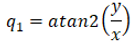Now lets see how we can derive q2 and q3 .

Give a close look at the robot from different angle, there is a triangle formed with link2 and link3 as show in the fig 8.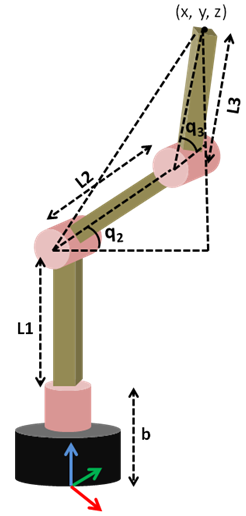Fig. 8 Side view of the manipulator

Now lets  take this triangles separately and label them as shown in fig 9.Fig. 9 Triangles drawn from fig 8 observation

Using triangle PRS shown in fig 10 and cosine rule we can find q3Cosine Rule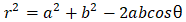Fig. 10 Triangles PRS to derive q3Using cosine rule we can write as below,Now we know q3 also. Finally the joint parameter left to find is q2.

From below fig we can writeFig. 11 Triangles to derive q2From right angle triangle PQS, we can get the value of    ,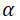From right angle triangle PST, we get the value of    ,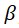Finally q2 is given byThat's it. We have found the equations to find the joint parameters q1, q2, q3 using geometric approach of our RRR spatial manipulator.

Lets write all equations for joint parameters below.Note: To solve IK for higher DOF robots using geometric approach then you should go with kinematic decoupling method. In this method first the manipulator is separated(decoupled) into small kinematic chains and IK is solved for those small chains. After finding equations for all joint parameters in kinematic chains, the IK problem (target Pose) is propagated from end-effector kinematic chain to base kinematic chain.

Thanks for reading. See you in next time.